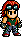# Armor King/Tekken 5: Dark Resurrection Movelist

Armor King/Tekken 5: Dark Resurrection Moves List is a list of all of the moves that Armor King can perform in Tekken 5: Dark Resurrection.Looks like Hwoarang has run off and made this page, Armor King/Tekken 5: Dark Resurrection Movelist, without telling Baek Doo San and hasn't done it right!Please fix it by re-formatting this page to follow it's [[Template:{{{1}}}|template]].

## §Moves List

1. One Two Punch: Square Triangle.
2. Jab Uppercut: Triangle Square.
3. Dark Cyclone: Square + Triangle Triangle.
4. Round Out Kick: X + Circle.
5. Round Out Body Press: X + Circle Square.
6. Round Out Elbow: X + Circle Triangle.
7. Dark Elbow Hook: East Triangle Square.
8. Switch-Kick Tornado: East X Circle X.
9. Wind-Up Stomp: East Circle.
10. Hammer Impact: East Square + Triangle.
11. Shoulder Impact: East Square + Circle.
12. Minced Liver: Southeast Square.
13. Concussion Hook: Southeast Triangle.
14. Dark Knee Lift: Southeast X.
15. Frankensteiner: Southeast X + Circle.
16. Knee Lift Combination: South Square (Neutral) Circle.
17. Corporate Elbow: South Square + Triangle.
18. Taurus Cutter: South X + Circle (or: (While crouching) Southeast Circle.).
19. Shadow Step: South Square + Circle (or South Triangle + X.).
20. Foot Sweep: Southwest X.
21. Low Drop Kick: Southwest Circle.
22. Dark Jab: West Square.
23. Blackjack: West Triangle.
24. Toll Kick: West X.
25. Reverse Switch-Kick Tornado: West Circle X.
26. Blindside: West Square + Triangle.
27. Straight Arrow: West Square + Circle.
28. Dark Destroyer: West Triangle + X.
29. Water Parting Chop: Northwest Square.
30. High Elbow Drop: Northeast Triangle.
31. Capital Punishment: Northeast Square + Triangle.
32. Burning Knuckle: Northeast Square + Triangle South (Hold the button down.).
33. Scissors Kick: Northeast X + Circle.
34. Jumping Capital Punishment: Northeast (Neutral) Square + Triangle.
35. Jumping Burning Knuckle: Northeast (Neutral) Square + Triangle South (Hold the button down.).
36. Jumping Stagger Kick: Northeast (Neutral) X + Circle.
37. Jumping Moonsault: Northeast (Neutral) Square + Circle.
38. Solar Plexus Punch: East East (Hold the button down) Triangle.
39. Comeback Kick: East East (Hold the button down) X.
40. Brawler Kick: East East (Hold the button down) Circle.
41. Flying Forearm: East East (Hold the button down) Square + Triangle.
42. Drop Kick: East East (Hold the button down) X + Circle.
43. Underhanded: East East (Neutral) Triangle.
44. Shadow Lariat: East (Neutral) South Southeast (Hold the button down) Square.
45. Palm Upper: East (Neutral) South Southeast (Hold the button down) Triangle.
46. Dark Inferno: East (Neutral) South Southeast (Hold the button down) X.
47. Knee Vault: East (Neutral) South Southeast (Hold the button down) Circle.
48. Poison Mist: East (Neutral) South Southeast (Hold the button down) Square + Triangle.
49. Flying Brawler Kick: East East East X.
50. Snap Uppercut: Square (While rising.).
51. Jugular Thrust: Triangle (While rising.).
52. Backhand Chop: Southeast Triangle (While crouching.).
53. Jaguar Thrust: Triangle (During sidestep.).
54. Buzz Saw Kick: Circle (During sidestep.).
55. Neck Cutter Kick: X + O (During sidestep.).
56. Blind Kick: X (Back towards enemy.).
57. Turn Sobat: Circle (Back towards enemy.).
58. Moonsault Drop: Square + Circle (Back towards enemy.).
59. 10 Hit Combo 1: Southeast Triangle Square X Triangle Triangle Circle Triangle Square X + Circle Triangle.
60. 10 Hit Combo 2: Southeast Triangle Square X Triangle Triangle X Circle Square + Triangle Triangle South Southeast East Square + Triangle.
61. Knee Bash: (Approach enemy) Square + X (or East Square + X.).
62. Suplex: (Approach enemy) Triangle + Circle (or East Triangle + Circle.).
63. Jaguar Screwdriver: (Approach enemy) Triangle + Circle (or East Triangle + Circle) South South South Square + Triangle.
64. Reverse Death Valley Bomb: (Approach from left side) Square + X (or Triangle + Circle.).
65. Heavy Fall Reverse DDT: (Approach from right side) Square + X (or Triangle + Circle.).
66. Reverse DDT: (Approach from behind) Square + X (or Triangle + Circle.).
67. DDT: (Approach enemy) Southwest Southwest Square + Triangle.
68. Tombstone Piledriver: (Approach enemy) Southwest East (Hold the button down) Triangle + Circle.
69. Double Arm Swing: (Approach enemy) East West Southwest South Southeast East Square. (or: (Approach enemy) East Northeast North Northwest West Southwest South Southeast East Square.).
70. Armor King Driver: Southwest South Southwest (Hold the button down) Square + Triangle (While crouching.).
71. Demon Bomb: (Approach (crouching enemy)) South Square + X.
72. Spinning Leg Lock: (Approach (crouching enemy)) South Triangle + Circle.
73. Brilliant Brawler Kick: East East East Triangle + Circle.
74. Mount Position: ((Enemy on back) by feet) Southwest Square + X (or Southwest Triangle + Circle.).
75. Turn Over: ((Enemy on back) by enemy's side) Southwest Square + X (or Southwest Triangle + Circle.).
76. Back Mount: (Enemy face down) Southwest Square + X (or Southwest Triangle + Circle.).
77. Muscle Driver: (Approach mid-air enemy) East West Southwest South Southeast East Square.
78. Running Cross Driver: (Approach mid-air enemy) East East East Triangle + Circle.

# Mexican Magma Drive #

1. Cobra Clutch: (Approach enemy) East (Neutral) South Southeast Square + Circle (or Triangle + X.).
2. Flinging Half Nelson: (During Cobra Clutch) Triangle + Circle Square + Triangle Square + Triangle.
3. Sleeper: (During Cobra Clutch) X + Circle X + Circle Square + Triangle.
4. Neck Drop: (During Cobra Clutch) Triangle Square Square + Triangle.
5. Triple Trouble: (During Sleeper) Square Square Square + Triangle.
6. Human Necktie: (During Sleeper) X Circle Square + Triangle X + Circle.
7. Choke Sleeper: (During Neck Drop) Circle X X + Circle Square + Triangle.
8. 3-Count Pound: (During Neck Drop) X + Circle Triangle Square Square Square + Triangle.